手把手教你生成对抗网络 GAN，50 行代码玩转 GAN 模型！

+关注继续查看

GAN，即生成对抗网络，主要包含两个模块：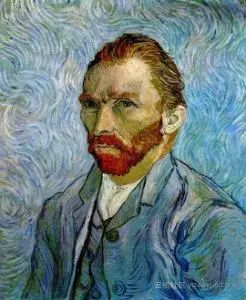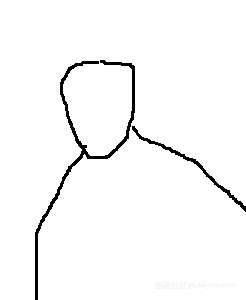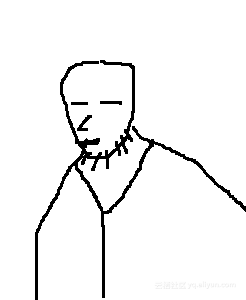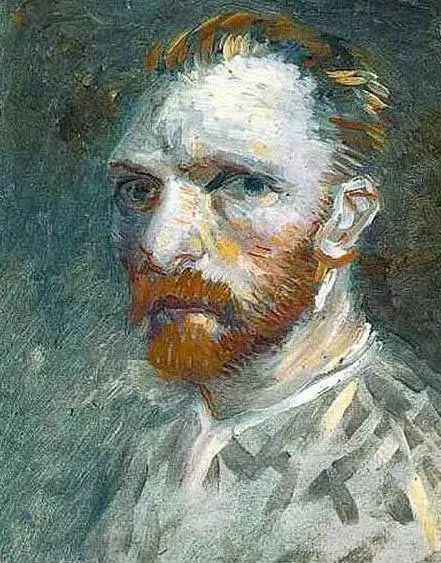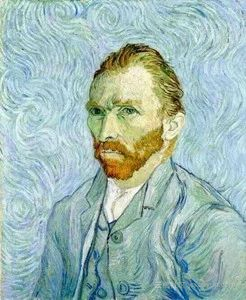GAN 模型基本结构

The most important one, in my opinion, is adversarial training (also called GAN for Generative Adversarial Networks). This is an idea that was originally proposed by Ian Goodfellow when he was a student with Yoshua Bengio at the University of Montreal (he since moved to Google Brain and recently to OpenAI).

This, and the variations that are now being proposed is the most interesting idea in the last 10 years in ML, in my opinion.

Yann LeCun 认为 GAN 很可能会给深度学习模型带来新的重大突破，是20年来机器学习领域最酷的想法。这几年 GAN 发展势头非常强劲。下面这张图是近几年 ICASSP 会议上所有提交的论文中包含关键词 “generative”、“adversarial” 和 “reinforcement” 的论文数量统计。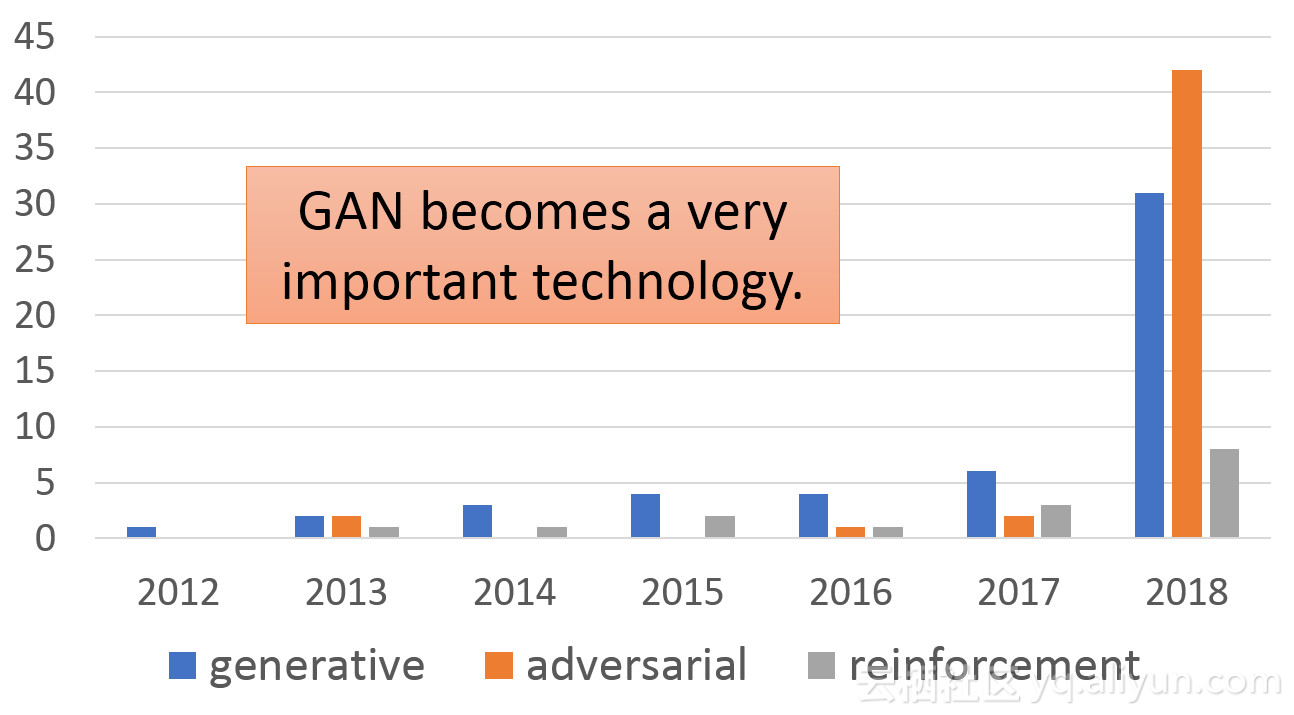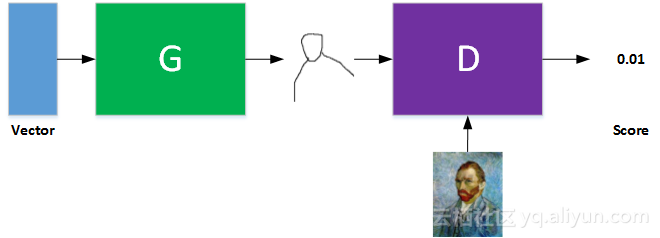GAN 基本模型由 输入 Vector、G 网络、D 网络组成。其中，G 和 D 一般都是由神经网络组成。G 的输出是一幅图片，只不过是以全连接形式。G 的输出是 D 的输入，D 的输入还包含真实样本集。这样， D 对真实样本尽量输出 score 高一些，对 G 产生的样本尽量输出 score 低一些。每次循环迭代，G 网络不断优化网络参数，使 D 无法区分真假；而 D 网络也在不断优化网络参数，提高辨识度，让真假样本的 score 有差距。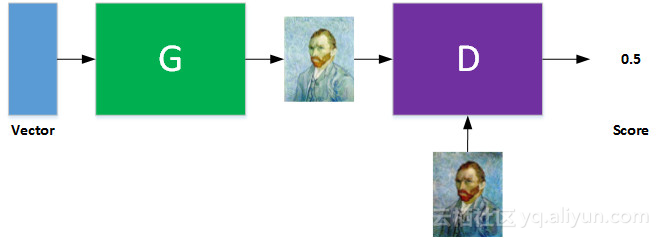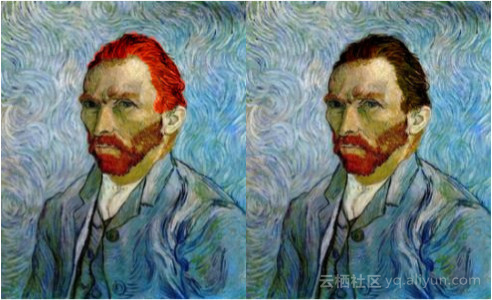GAN 的强大之处也正是在于此，通过调节输入 vector，就可以生成具有不同特征的图片。而这些生成的图片不是真实样本集里有的，而是即合理而又没有见过的图片。是不是很有意思呢？下面这张图反映的是不同的 vector 生成不同的图片。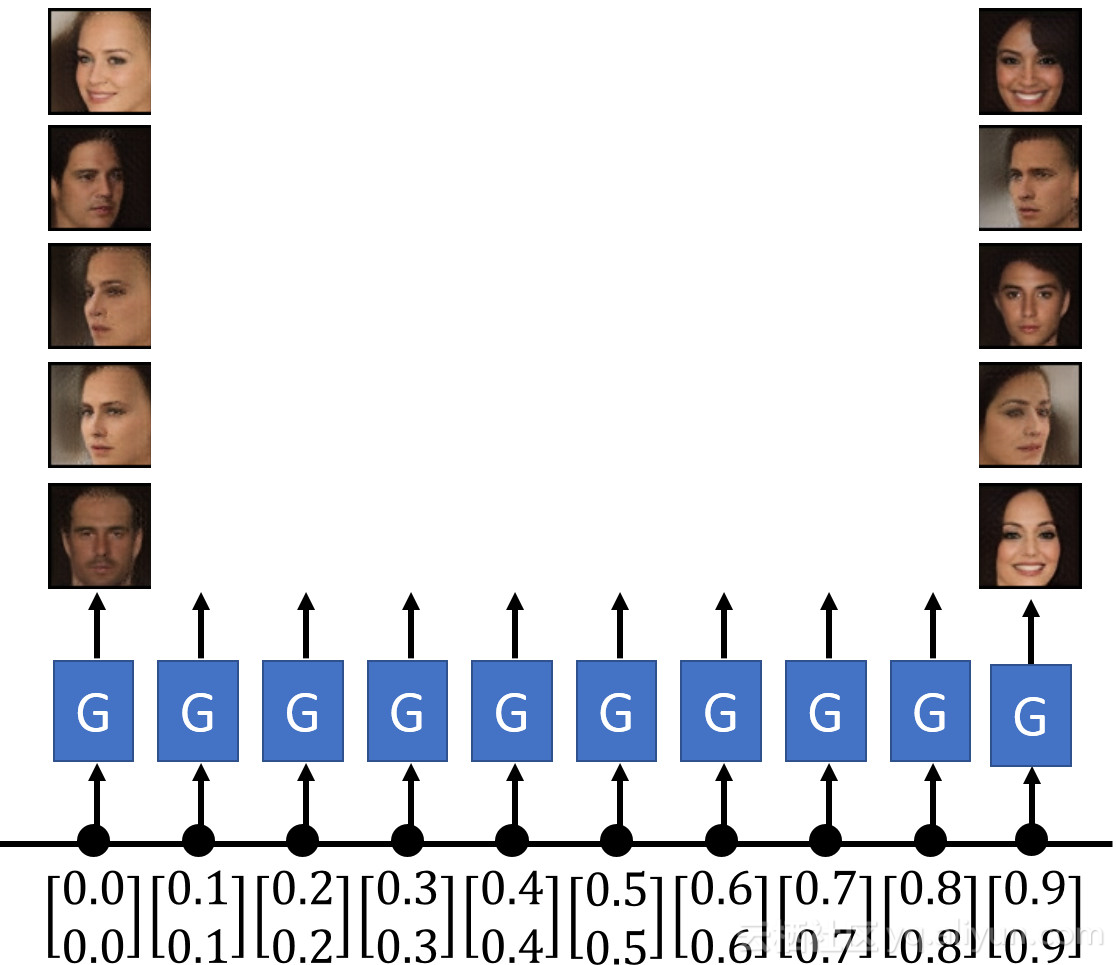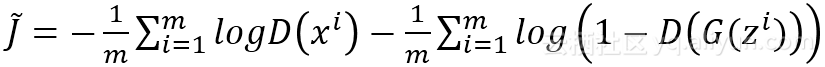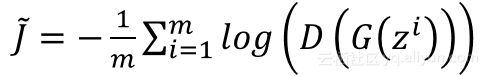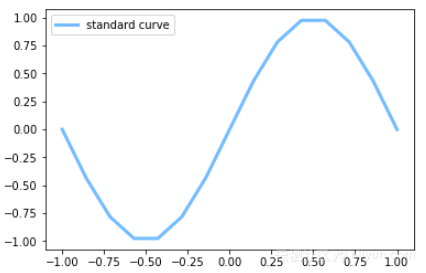def artist_works():    # painting from the famous artist (real target)
r = 0.02 * np.random.randn(1, ART_COMPONENTS)
paintings = np.sin(PAINT_POINTS * np.pi) + r
paintings = torch.from_numpy(paintings).float()
return paintings

G = nn.Sequential(                  # Generator
nn.Linear(N_IDEAS, 128),        # random ideas (could from normal distribution)
nn.ReLU(),
nn.Linear(128, ART_COMPONENTS), # making a painting from these random ideas
)

D = nn.Sequential(                  # Discriminator
nn.Linear(ART_COMPONENTS, 128), # receive art work either from the famous artist or a newbie like G
nn.ReLU(),
nn.Linear(128, 1),
nn.Sigmoid(),                   # tell the probability that the art work is made by artist
)

plt.ion()    # something about continuous plotting

D_loss_history = []
G_loss_history = []
for step in range(10000):
artist_paintings = artist_works()          # real painting from artist
G_ideas = torch.randn(BATCH_SIZE, N_IDEAS) # random ideas
G_paintings = G(G_ideas)                   # fake painting from G (random ideas)

prob_artist0 = D(artist_paintings)         # D try to increase this prob
prob_artist1 = D(G_paintings)              # D try to reduce this prob

D_loss = - torch.mean(torch.log(prob_artist0) + torch.log(1. - prob_artist1))
G_loss = torch.mean(torch.log(1. - prob_artist1))

D_loss_history.append(D_loss)
G_loss_history.append(G_loss)

D_loss.backward(retain_graph=True)    # reusing computational graph
opt_D.step()

G_loss.backward()
opt_G.step()

if step % 50 == 0:  # plotting
plt.cla()
plt.plot(PAINT_POINTS, G_paintings.data.numpy(), c='#4AD631', lw=3, label='Generated painting',)
plt.plot(PAINT_POINTS, np.sin(PAINT_POINTS * np.pi), c='#74BCFF', lw=3, label='standard curve')
plt.text(-1, 0.75, 'D accuracy=%.2f (0.5 for D to converge)' % prob_artist0.data.numpy().mean(), fontdict={'size': 8})
plt.text(-1, 0.5, 'D score= %.2f (-1.38 for G to converge)' % -D_loss.data.numpy(), fontdict={'size': 8})
plt.ylim((-1, 1));plt.legend(loc='lower right', fontsize=10);plt.draw();plt.pause(0.01)

plt.ioff()
plt.show()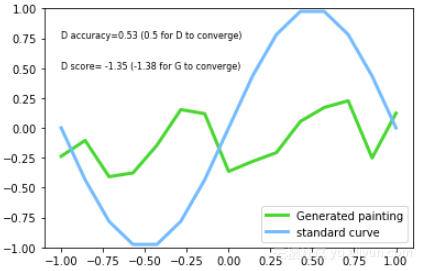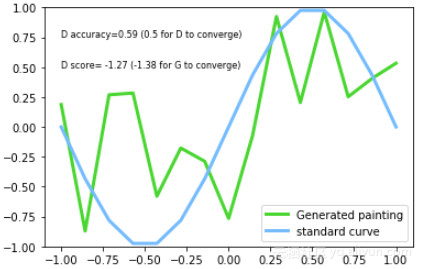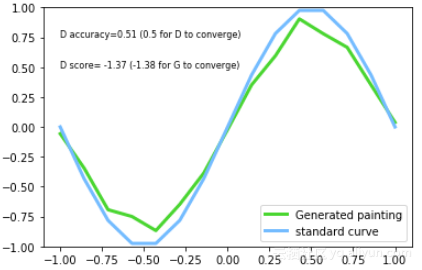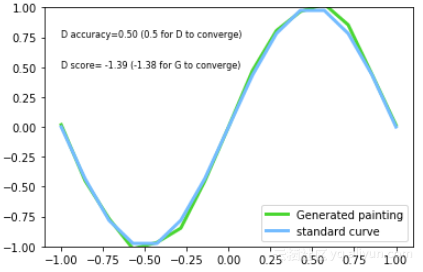MyBatis Generator 代码生成器 快速入门指南

805 01624 0804 02027 09315 07527 0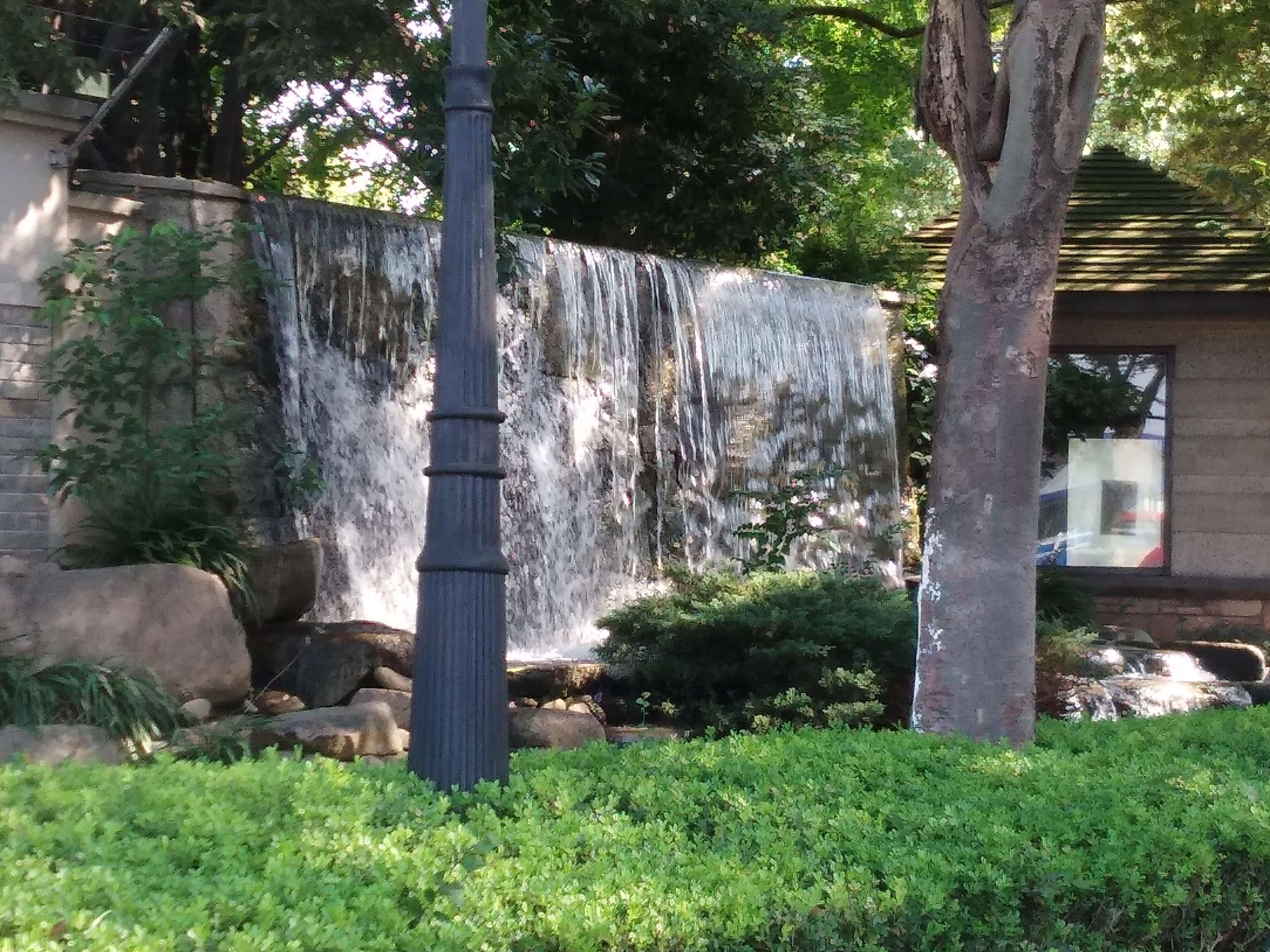05.Java网络编程（代码实践）

783 04459 0699 0GAN网络通俗解释（图画版）

4996 0

7208

9

+ 订阅

《2021云上架构与运维峰会演讲合集》

《零基础CSS入门教程》

《零基础HTML入门教程》